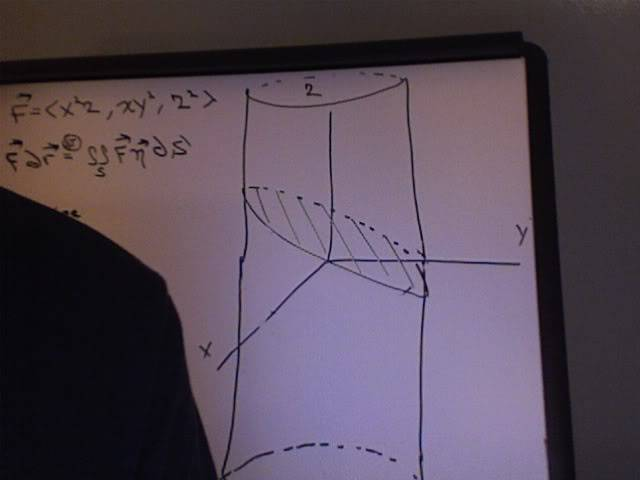# Stoke's Theorem

## Homework Statement

Use Stoke's Theorem to calculate $\int_C\vec{F}\cdot\, dr$, where
$\vec{F}=<x^2z\, ,xy^2\,,z^2>$ and C is the curve of the intersection of the plane $x+y+z=1$ and the cylinder
$x^2+^2=9$.
(C is oriented clockwise when viewed from above.)
Answer: $\frac{81}{2}\pi$.

Stoke's Theorem

## The Attempt at a Solution

Okay, let me try to explain where I am getting lost. Firstly, I know that the premise of Stoke's Theorem is that is relates a line integral to a Surface integral.

When I graph this, I get a cylinder that is symmetrical about the z-axis and it is intersected by a plane which results in an ellipse. (see terrible drawing below)

Now, I need to parametrize (how do you spell that anyway?) S. Now S is the surface that is bounded by C right? If not, please stop me here.Defennder
Homework Helper
Now, I need to parametrize (how do you spell that anyway?) S. Now S is the surface that is bounded by C right? If not, please stop me here.
Yes. S is any surface that is bounded by C. Usually the easiest surface to work with is the plane surface bounded by C if C can be visualised as lying on a plane.

Yes. S is any surface that is bounded by C. Usually the easiest surface to work with is the plane surface bounded by C if C can be visualised as lying on a plane.

I know form doing this in class that we parametrized S as r=<x, y, 1-x-y>

but I am not so sure why. Oh wait... is that the surface bounded by the ellipse?

So now all I need to do is find dr/dx x dr/dy and compute the dot product of the resultant with F(r(x,y) and integrate?

You are told that x + y + z = 1 and now you parametrize that by <x,y,f(x,y)> and z = f(x,y) = 1 - x - y by algebra (is that part of your question?)

I guess my question is what is S? Is it the the surface of the ellipse? I believe it is, so I guess my question has been answered.